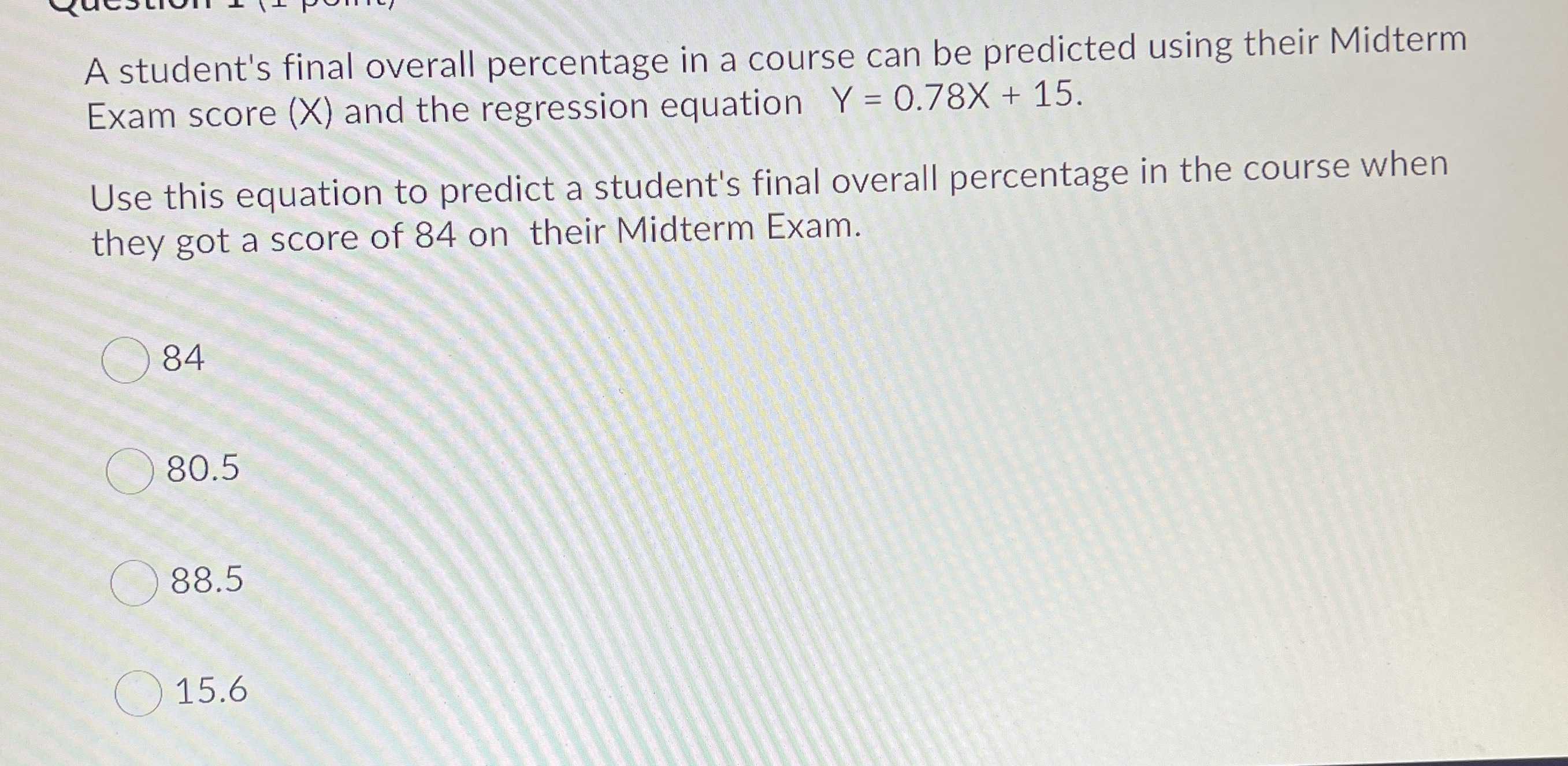### Still have math questions?

Algebra
QuestionA student's final overall percentage in a course can be predicted using their Midterm Exam score $$( X )$$ and the regression equation $$Y = 0.78 X + 15$$ . Use this equation to predict a student's final overall percentage in the course when they got a score of $$84$$ on their Midterm Exam.

(A) $$84$$

(B) $$80.5$$

(C) $$88.5$$

(D) $$15.6$$